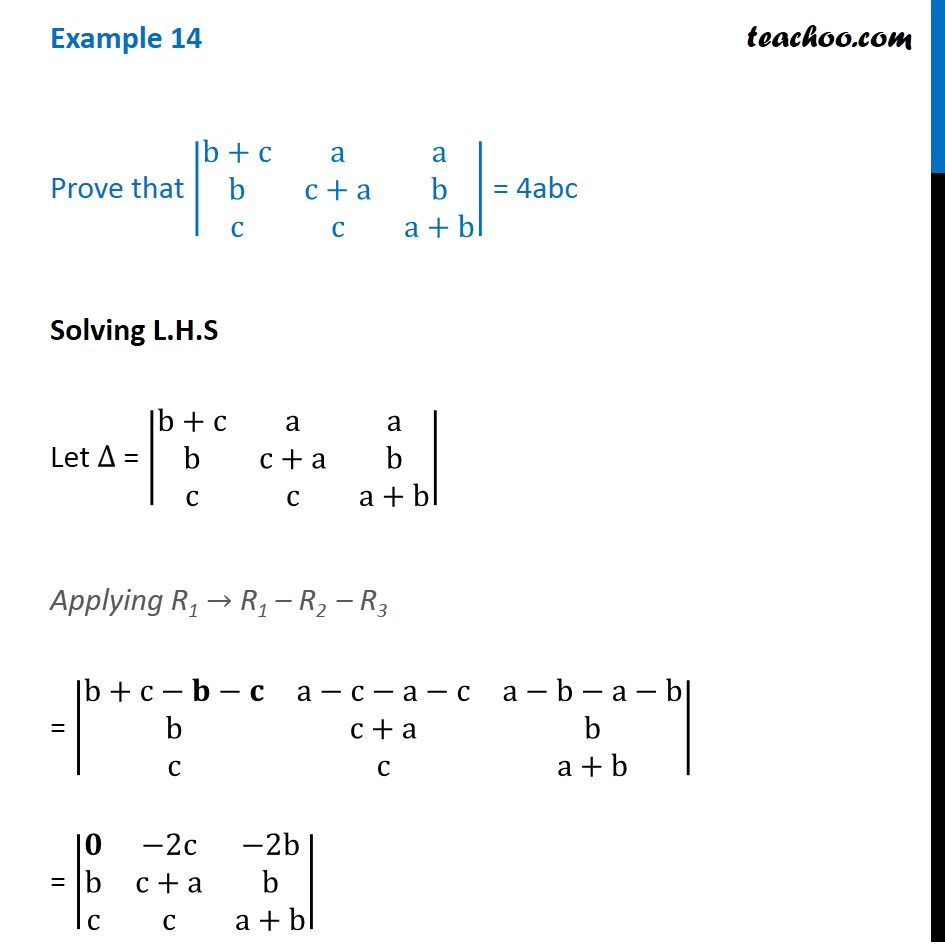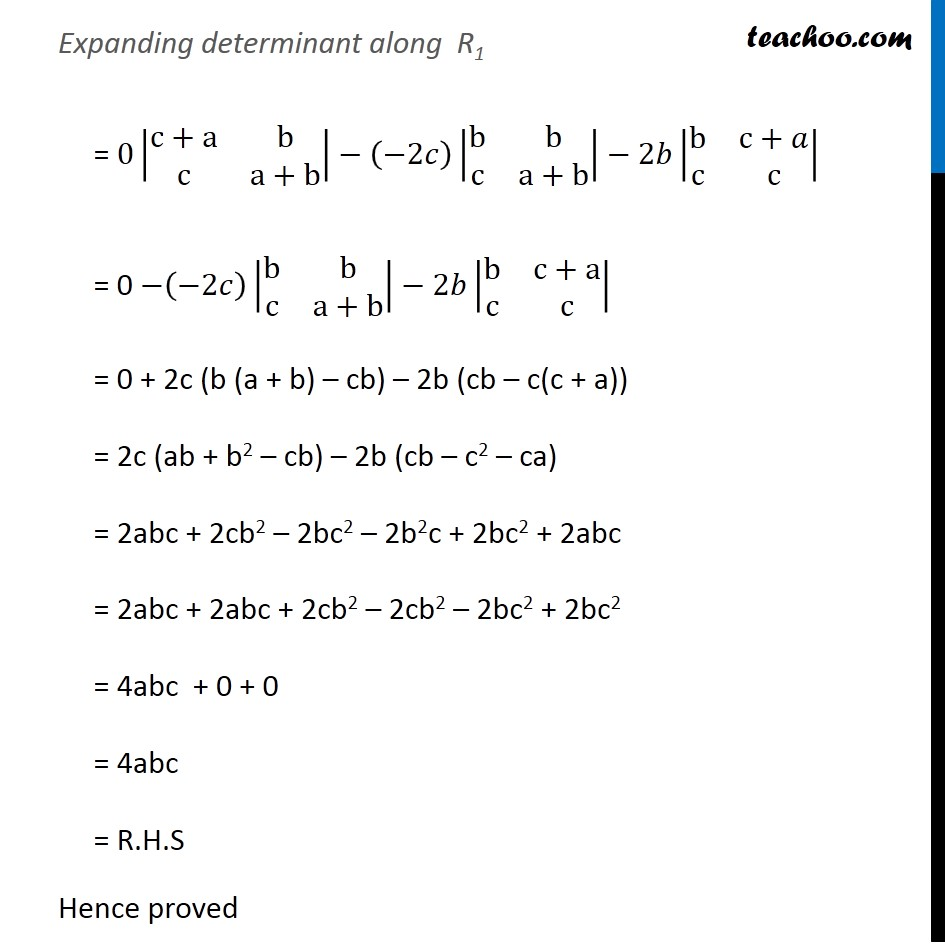Examples

Chapter 4 Class 12 Determinants
Serial order wiseLearn in your speed, with individual attention - Teachoo Maths 1-on-1 Class

### Transcript

Question 9 Prove that |■8(b+c&a&[email protected]&c+a&[email protected]&c&a+b)| = 4abc Solving L.H.S Let ∆ = |■8(b+c&a&[email protected]&c+a&[email protected]&c&a+b)| Applying R1 → R1 – R2 – R3 = |■8(b+c−𝐛−𝐜&a−c−a−c&a−b−a−[email protected]&c+a&[email protected]&c&a+b)| = |■8(𝟎&−2c&−[email protected]&c+a&[email protected]&c&a+b)| Expanding determinant along R1 = 0|■8(c+a&[email protected]&a+b)|−(−2𝑐)|■8(b&[email protected]&a+b)|−2𝑏|■8(b&c+𝑎@c&c)| = 0 −(−2𝑐)|■8(b&[email protected]&a+b)|−2𝑏|■8(b&[email protected]&c)| = 0 + 2c (b (a + b) – cb) – 2b (cb – c(c + a)) = 2c (ab + b2 – cb) – 2b (cb – c2 – ca) = 2abc + 2cb2 – 2bc2 – 2b2c + 2bc2 + 2abc = 2abc + 2abc + 2cb2 – 2cb2 – 2bc2 + 2bc2 = 4abc + 0 + 0 = 4abc = R.H.S Hence proved